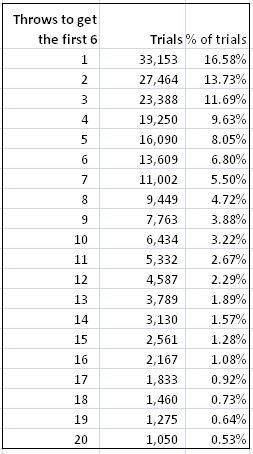# Guessing the number of throws required to get a 6 on a fair die

• musicgold

#### musicgold

Homework Statement
Not a homework problem. I wanted to find out what is the best guess for the number of throws required to get a 6 on a dice.
Relevant Equations
So I ran a simulation and the results seem counterintutive. See the results below.
The table below shows the probabilities for getting a 6 in up to 20 throws.

1. I know that the expected number of throws is 6, but that number doesn't seem to be a useful guide here, even in the long run. 1 throw seems to be the best guess. What am I missing?

2. The cumulative probability of getting a 6, crosses the 50% mark at around 4. How do we reconcile that fact with the expected number of throws of 6?Thanks

The mode, or single most likely outcome, is you get it in the first throw. It matters a lot what you mean when you say "best guess". If you get paid a dollar for guessing exactly how many rolls it takes then you should guess 1. But that's not typically what people mean when they say best guess, they usually mean make a guess which on average is as close as possible, e.g minimizing the sum of the magnitude of your errors.

Part 2 is known as the median, and it's also a useful thing you think about sometimes. But suppose you and I gamble. I will pay you five dollars, then you pay me $1 for every roll required to get a six. Even though you will make money more often than I will, when I do make money I will make more of it than you. A lot of the time you will make$1, but sometimes I make \$6 and that overwhelms your small quantities over repeated play.

•FactChecker
I'm not getting the "counterintuitive" part. Are you, perhaps, confusing rolling the first 6 on the 13th throw with rolling a 6 on any of the first 13 throws?

•PeroK
Homework Statement:: Not a homework problem. I wanted to find out what is the best guess for the number of throws required to get a 6 on a dice.
Relevant Equations:: So I ran a simulation and the results seem counterintutive. See the results below.

The table below shows the probabilities for getting a 6 in up to 20 throws.

1. I know that the expected number of throws is 6, but that number doesn't seem to be a useful guide here, even in the long run. 1 throw seems to be the best guess. What am I missing?

2. The cumulative probability of getting a 6, crosses the 50% mark at around 4. How do we reconcile that fact with the expected number of throws of 6?
1) If you're betting on when the first six will come up, then your best chance is the first throw. Because all you need is to throw a six on the first throw. To get your first six on the nth throw you need ##n-1## throws that are not six and a six on the nth throw. Hence the probabilities reduce quickly, as we can see from your data.

2) You reconcile it by calculating calculating the expected number of throws. Note that expected value has a precise meaning: $$E = \sum_{n = 1}^{\infty}np(n)$$ That's very different from the mode, which is the most common and in this case is ##1##, and the median which is the midpoint of a distribution and in this case is ##4##. The expected value is also known as the mean. If "expected value" carries too much linguistic baggage for you, then sick to using mean.

•Frabjous and musicgold